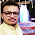## June 09, 2013

### Reversible Logic Gates

Who would have thought that even digital logic gates could be reversible in nature? Well, as it turns out, there's been a lot of research already done on the subject. In this post, I would only discuss the motivation behind building reversible logic gates.

Every node can be generalized as a capacitance that would undergo charging/discharging depending upon the inputs and the logic that that particular gate has been designed to realize. And every node would dissipate charge in form of leakage power. What if someone takes the output and feeds it back to the inputs forming a "Positive Feedback" structure! In order words, one can sustain the inputs from outputs and outputs would anyway be dependent on inputs. Doing so can save a lot of leakage power and this exactly is the motivation behind building reversible logic gates. Cool, isn't it? Have a look below where C is any combinatorial logic gate.

It might be easier to appreciate the advantage of such a logic gate when many of them are attached in cascade as shown below. Here the output of one gate would sustain it's input which, in turn, would be the output of some other logic gate and it would continue on forever!!

Enough said. Here's the paper: Efficient Building Blocks for Reversible Sequential Circuit Design by Hari, Shroff, Mahammad & Kamakoti which you can read to understand the implementation better. Please let me know in case you come across any work related to "Reversible Logic Gates".

#### 1 comment:

1.Hello Sir I want your guidance